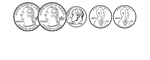62 Cents

Groups of change with totals from 1 to 100 cents using the least amount of coins.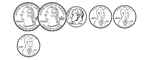63 Cents

Groups of change with totals from 1 to 100 cents using the least amount of coins.64 Cents

Groups of change with totals from 1 to 100 cents using the least amount of coins.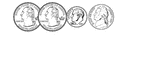65 Cents

Groups of change with totals from 1 to 100 cents using the least amount of coins.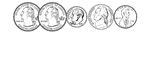66 Cents

Groups of change with totals from 1 to 100 cents using the least amount of coins.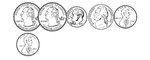67 Cents

Groups of change with totals from 1 to 100 cents using the least amount of coins.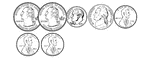68 Cents

Groups of change with totals from 1 to 100 cents using the least amount of coins.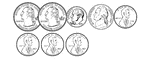69 Cents

Groups of change with totals from 1 to 100 cents using the least amount of coins.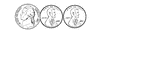7 Cents

Groups of change with totals from 1 to 100 cents using the least amount of coins.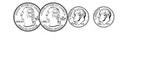70 Cents

Groups of change with totals from 1 to 100 cents using the least amount of coins.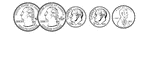71 Cents

Groups of change with totals from 1 to 100 cents using the least amount of coins.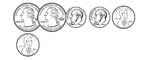72 Cents

Groups of change with totals from 1 to 100 cents using the least amount of coins.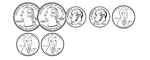73 Cents

Groups of change with totals from 1 to 100 cents using the least amount of coins.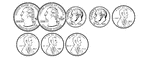74 Cents

Groups of change with totals from 1 to 100 cents using the least amount of coins.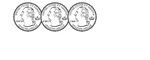75 Cents

Groups of change with totals from 1 to 100 cents using the least amount of coins.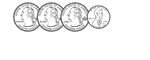76 Cents

Groups of change with totals from 1 to 100 cents using the least amount of coins.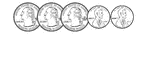77 Cents

Groups of change with totals from 1 to 100 cents using the least amount of coins.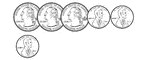78 Cents

Groups of change with totals from 1 to 100 cents using the least amount of coins.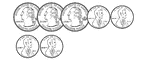79 Cents

Groups of change with totals from 1 to 100 cents using the least amount of coins.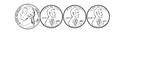8 Cents

Groups of change with totals from 1 to 100 cents using the least amount of coins.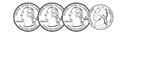80 Cents

Groups of change with totals from 1 to 100 cents using the least amount of coins.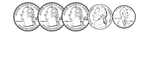81 Cents

Groups of change with totals from 1 to 100 cents using the least amount of coins.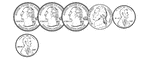82 Cents

Groups of change with totals from 1 to 100 cents using the least amount of coins.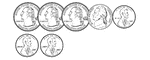83 Cents

Groups of change with totals from 1 to 100 cents using the least amount of coins.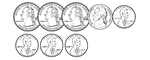84 Cents

Groups of change with totals from 1 to 100 cents using the least amount of coins.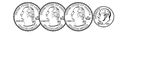85 Cents

Groups of change with totals from 1 to 100 cents using the least amount of coins.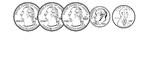86 Cents

Groups of change with totals from 1 to 100 cents using the least amount of coins.87 Cents

Groups of change with totals from 1 to 100 cents using the least amount of coins.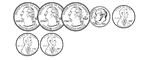88 Cents

Groups of change with totals from 1 to 100 cents using the least amount of coins.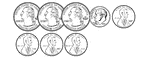89 Cents

Groups of change with totals from 1 to 100 cents using the least amount of coins.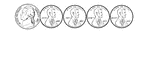9 Cents

Groups of change with totals from 1 to 100 cents using the least amount of coins.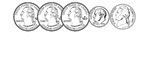90 Cents

Groups of change with totals from 1 to 100 cents using the least amount of coins.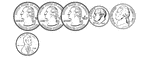91 Cents

Groups of change with totals from 1 to 100 cents using the least amount of coins.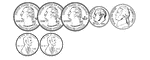92 Cents

Groups of change with totals from 1 to 100 cents using the least amount of coins.93 Cents

Groups of change with totals from 1 to 100 cents using the least amount of coins.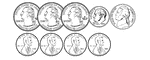94 Cents

Groups of change with totals from 1 to 100 cents using the least amount of coins.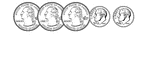95 Cents

Groups of change with totals from 1 to 100 cents using the least amount of coins.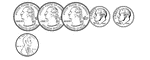96 Cents

Groups of change with totals from 1 to 100 cents using the least amount of coins.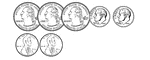97 Cents

Groups of change with totals from 1 to 100 cents using the least amount of coins.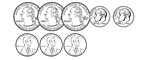98 Cents

Groups of change with totals from 1 to 100 cents using the least amount of coins.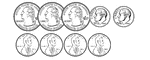99 Cents

Groups of change with totals from 1 to 100 cents using the least amount of coins.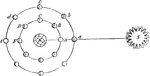Moon Phases

"Let S be the Sun, E the Earth, and A, B, C, D, F, the Moon in different parts of her orbit. Now when…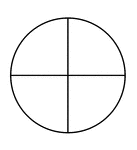Fraction Pie Divided into Quarters

A circle divided into quarters.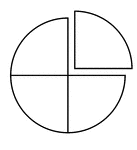Fraction Pie Divided into Quarters

A circle divided into quarters with one quarter separated.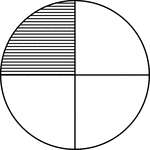Fraction Pie Divided into Quarters

A circle divided into quarters with one quarter shaded.Fraction Pie Divided into Quarters

A circle divided into quarters with two quarters shaded.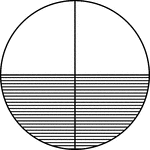Fraction Pie Divided into Quarters

A circle divided into quarters with two quarters shaded.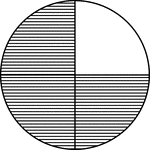Fraction Pie Divided into Quarters

A circle divided into quarters with three quarters shaded.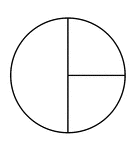One Half and Two Quarters of a Pie Fraction

A circle subdivided into one half and two quarters.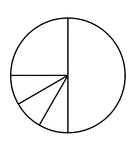One Quarter, Three Twelfths, and One Half of a Pie Fraction

A circle subdivided into one quarter, three twelfths, and one half.One Quarter, Two Eighths, and One Half of a Pie Fraction

A circle subdivided into one quarter, two eighths, and one half.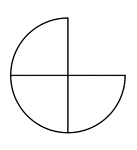Three Quarters of a Fraction Pie

Three quarters of a circle.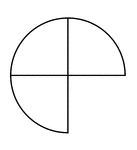Three Quarters of a Fraction Pie

Three quarters of a circle.Three Quarters of a Fraction Pie

Three quarters of a circle.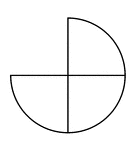Three Quarters of a Fraction Pie

Three quarters of a circle.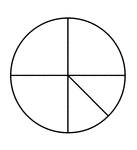Three Quarters and Two Eighths of a Pie Fraction

A circle subdivided into three quarters and two eighths.